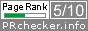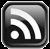# LEARN MS-ACCESS TIPS AND TRICKS

Learn Microsoft Access Advanced Programming Techniques, Tips and Tricks.

### Introduction.

How to find the week-end date or first-day date of a week using Current Date as input.  Or find first/last-day date of any week by giving a date value as input.

These kind of calculations may become necessary to find week-end date and use it as criteria in queries, filter data for viewing or printing of Reports for sharing of information.

Wherever you need it you can use the following simple expression to find the week-end date of current week:

### The Simple Expressions

```LastDayOfWeek = Date()-WeekDay(date())+7
```

If current date is 14th June, 2017 (or any date between 11 and 17 June 2017) then the value returned in variable LastDayOfWeek = 17th June, 2017.

#### Exaple-1

To find the first-day date of the current week use the following method:

```FirstDayOfWeek = date()-WeekDay(date())+1
```

Assuming current date is 14th June, 2017 (or any date between 11 and 17 June 2017) the first-day date of the week returned in variable FirstDayofWeek = 11th June, 2017.

#### Exaple-2

By giving a specific date as input to the expression to find the last-day date of the week:

```dtValue = #06/27/2017#
LastDayOfWeek = dtValue - WeekDay(dtValue)+7
Result: 1st July 2017
```

#### Exaple-3

If you would like to do it differently then try the following expression:

```x = #06/27/2017#
'To find the last-day date of the Week:
LastDayofWeek = DateSerial(Year(Date()),1,1)+DatePart("ww",x)*7-1
Result: 1st July, 2017
'To find the first-day date of the Week.
FirstDayofWeek = DateSerial(Year(Date()),1,1)+DatePart("ww",x)*7-7
Result: 25th June, 2017
```

### WeekLastDay Function

Define it as a Function in a VBA Module and call it wherever you want it, with a date value as parameter. Sample Function code is given below:

```Public Function WeekLastDay(ByVal dtVal As Date) As Date
'Date value as input
WeekLastDay = dtVal - WeekDay(dtVal) + 7
End Function
```

### WeekFirstDay Function

```Public Function WeekFirstDay(ByVal dtVal As Date) As Date
'Date value as input
WeekFirstDay = dtVal - WeekDay(dtVal) + 1
End Function
```
Share:

## TranslateSubscribe in a readerYour email address:

Delivered by FeedBurner